# 在Visual Studio中开发Matlab mex文件，生成mexw64/mexw32

csunking贡献，2015-9-22 1712

# 2、   步骤

1.启动VS，新建一个空的项目ATSRID。然后配置项目属性信息。然后，新建一个.cpp文件atsrid.cpp。并在添加Preprocessor Definitions中添加MATLAB_MEX_FILE的宏定义。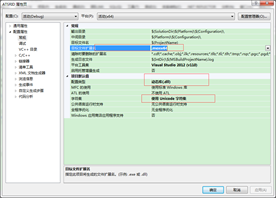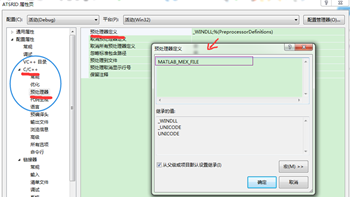$(OutDir)$(TargetName).mexw64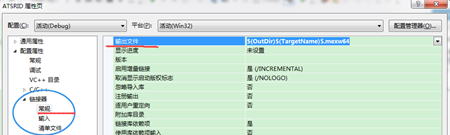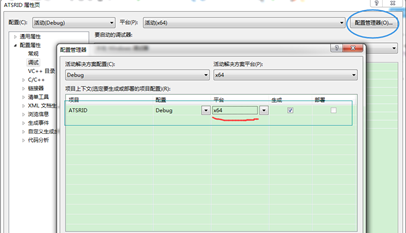2. 为matlab函数提供头文件和静态库的支持

E:\Program Files\MATLAB\R2015a\extern\include

E:\Program Files\MATLAB\R2015a\extern\include\win64

E:\Program Files\MATLAB\R2015a\extern\lib\win64\microsoft

libmx.lib;libmat.lib;libmex.lib;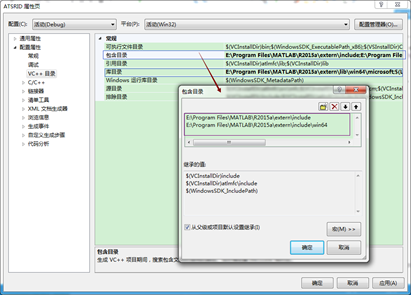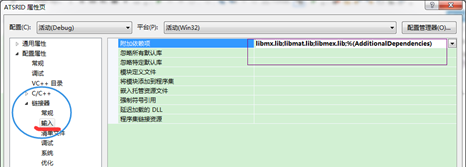3. 在atsrid.cpp中编写mex文件中的内容。然后用利用.def文件导出mexFunction函数。

#include "mex.h"

void mexFunction(int nlhs,mxArray *plhs[],int nrhs,const mxArray *prhs[])
{
int nlhs1,nrhs1;
mxArray *plhs1,*prhs1;
mxArray *array_ptr;

nlhs1=1;
nrhs1=1;

if((array_ptr=mexGetVariable("base","x"))==NULL)   //"base" means the command window where
{                                                   //we get the matrix "x".
mexErrMsgTxt("Variable 'x' not in the workspace.");
}
else
{
prhs1=array_ptr;
mexCallMATLAB(nlhs1,plhs1,nrhs1,prhs1,"det");
mexPrintf("Det(array_ptr) == %g\n",mxGetScalar(plhs1));
}

}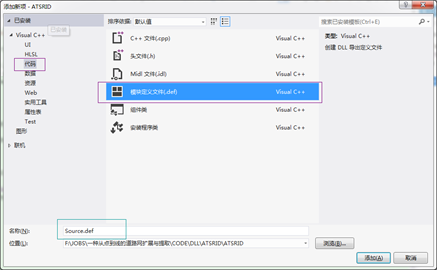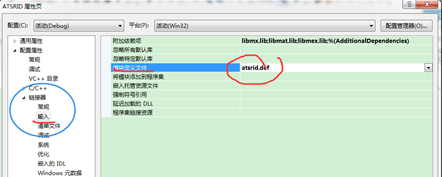LIBRARY ATSRID; 指的是模块名称（可以用新建的项目名称）

EXPORTS mexFunction

4. 编译运行，生成ATSRID.mexw64文件。然后将该文件拷贝到Matlab的当前目录下，然后进行该模块的测试
x=magic(100);

ATSRID();

# 3、  采用VS Debug mex文件

1、初次使用mex时需要配置配置matlab mex编译器

mex -setup

2、接下来，用-g选项编译mex源文件，-g表示编译结果中包含调试信息。

mex -g atsrid.cpp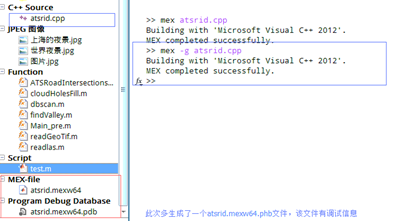3、开启Visual Studio，不要关闭你的matlab。在Visual Studio的工具菜单中，选择关联到一个进程。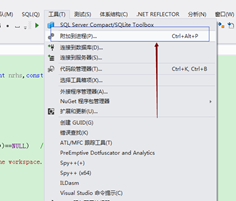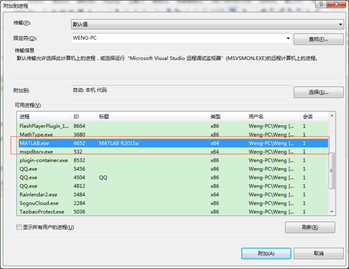Visual Studio会加载数据，在弹出的对话框中选择，选择matlab进程，点击“关联”。

4、首先在Visual Studio的ATSRID项目中的atsrid.cpp文件代码处添加断点。然后，打开matlab 放置atsrid.mexw64的当前目录，在代码行中输入下面的代码

x=magic(10);

atsrid();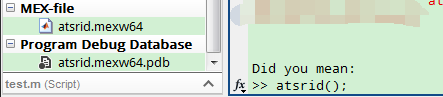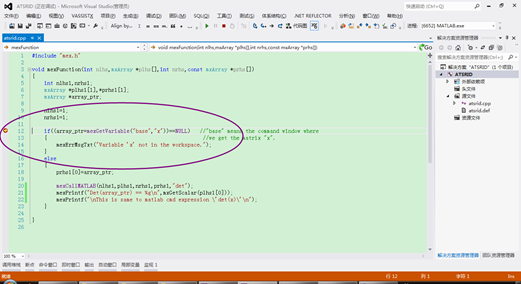posted @ 2016-01-05 21:10  wenglabs  阅读(...)  评论(...编辑  收藏